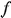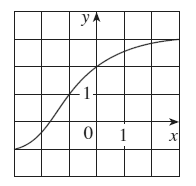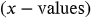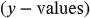1
37
views
18b
Problem

Problem 18b

Chapter 1: Functions and ModelsTextbook ExpertVerified Tutor
13 Nov 2021

Given information

The graph of the functionisStep-by-step explanation

Step 1.

The domain is the set of all possible inputsfor which the function is defined.

The range is the set of defined output values.

The domain of an inverse function is the range of the original, and the range of an inverse function is the domain of an original function.